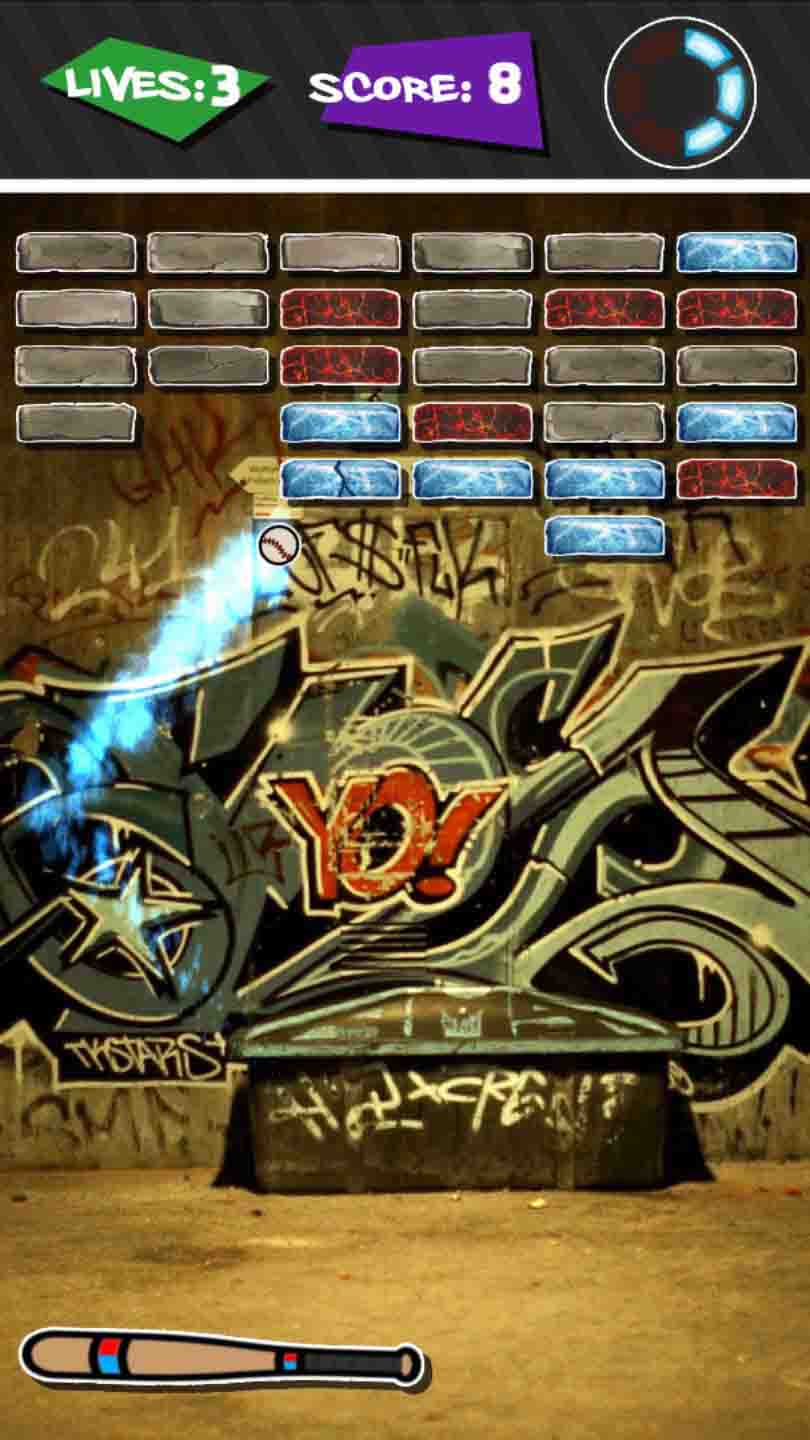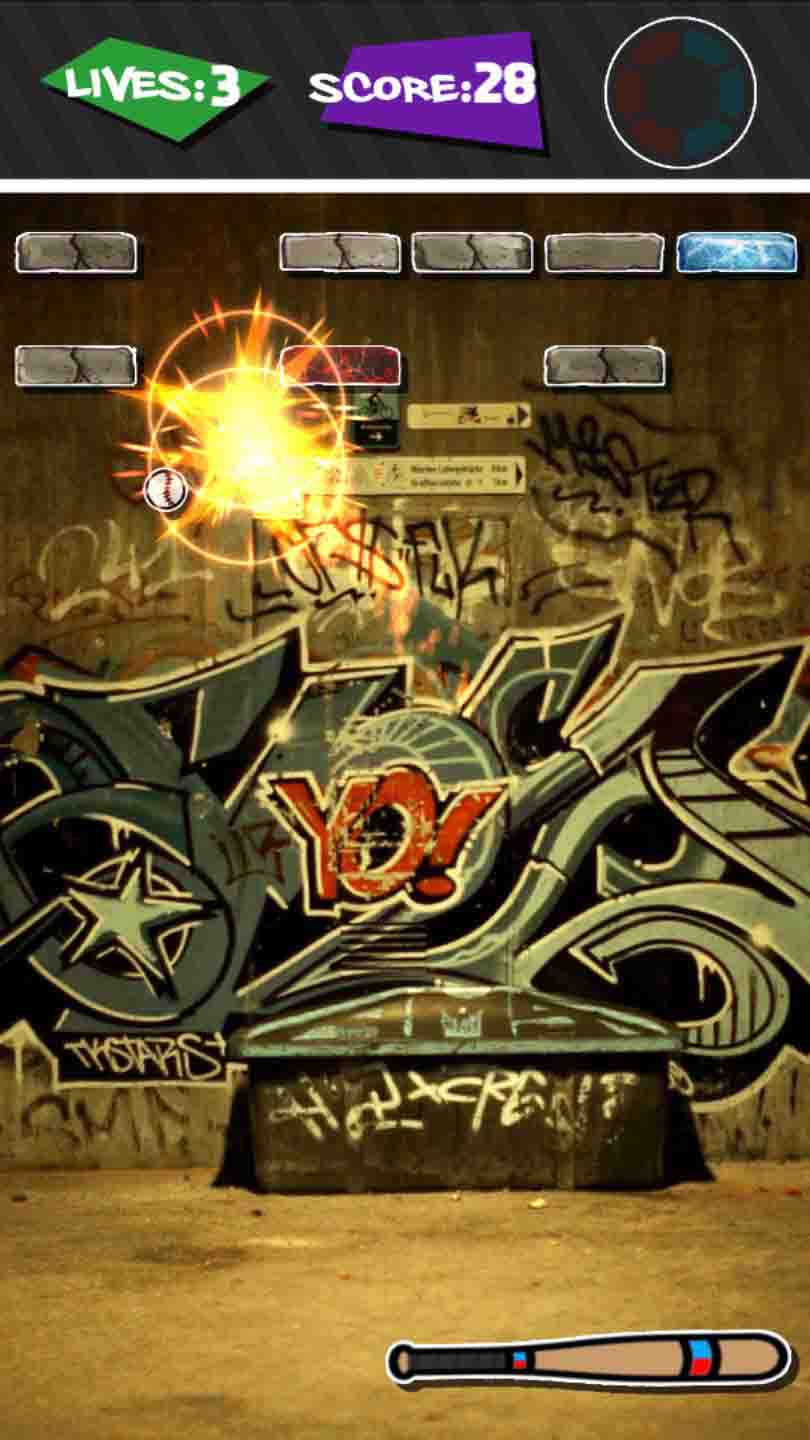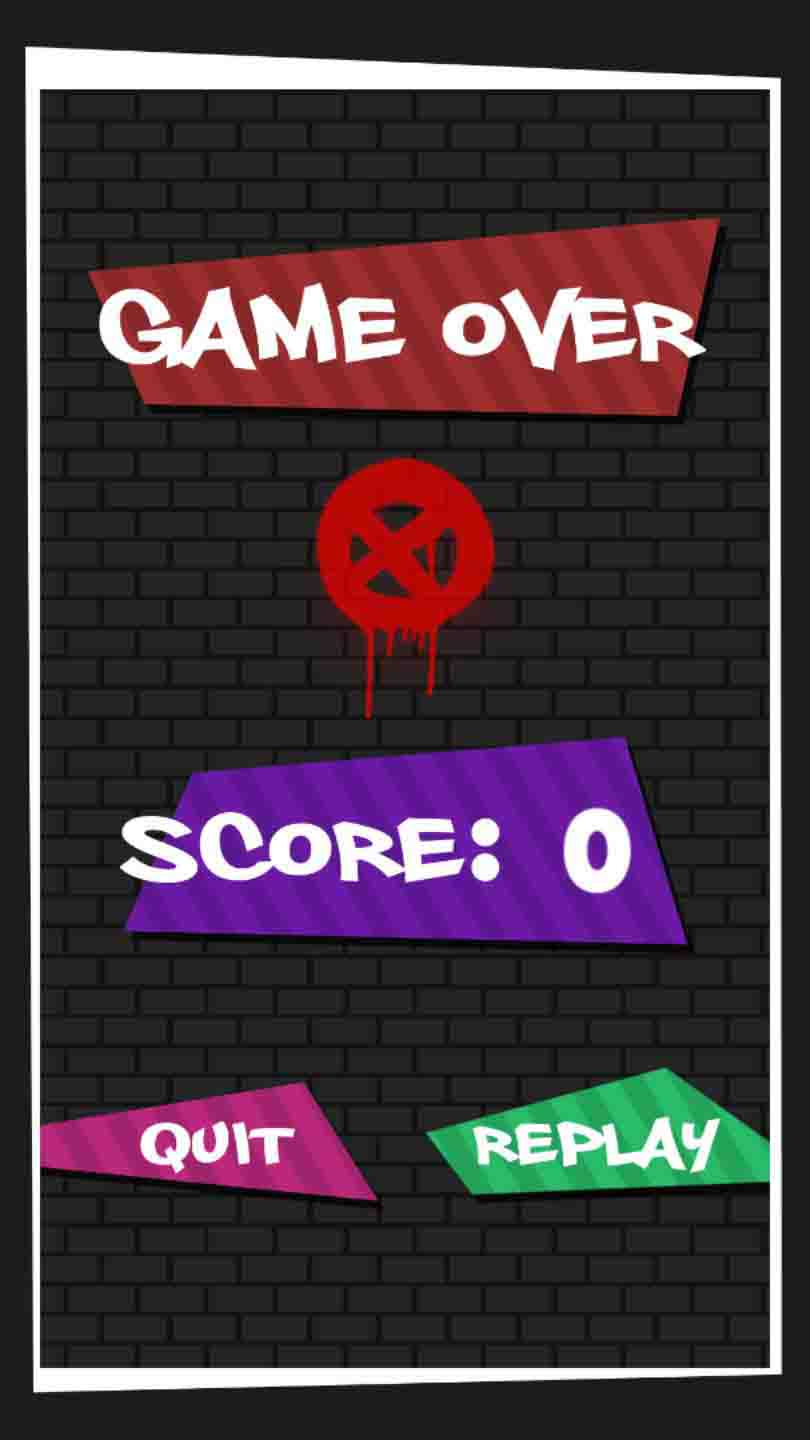## Breakout Urban

C#
Unity
⛋ 2D
Uni
06/2018

My first Unity project, made with the help of Trent Williams and Tristan Bondici for a University project. This is a small game made in the space of approximately 6 weeks.

This game uses the core mechanics of Breakout with the added twist that the paddle is now a baseball bat and the ball has the ability to heat up or cool down based on which side of the bat it hits and what bricks it hits. At max-cold or max-heat, the ball will cause either a row or a column of bricks to be destroyed, respectively. The layout of the bricks is entirely randomised with each play-through.

Up until recently, this had no plans for further work or release beyond on itch.io. This has now changed, and I have taken it upon myself to use this game as a way to explore the Google Play Store app pipeline. I have spent about a month reworking a lot of the logic to run more efficiently and I am nearing release soon.

### Team:

Liam Dunstan - Designer, Programmer
Trent Williams - Designer, Artist, Secondary Programmer
Tristan Bondici - Designer, Sound Guy

1 / 72 / 73 / 74 / 75 / 76 / 77 / 7### Code Snippets

```                                ```void CreateBricks()
{
// first brick y-pos
float y = 7.16f;
// create parent object
Transform bricks = new GameObject().transform;

for (int i = 0; i < 6; i++)
{
// first brick x-pos
float x = -4.06f;
for (int j = 0; j < 6; j++)
{
// create brick and add necessary components
Transform brick = new GameObject().transform;
brick.parent = bricks;
brick.position = new Vector3(x, y, 0);
brick.gameObject.AddComponent<SpriteRenderer>();
brick.gameObject.AddComponent<BrickScript>();
brick.gameObject.AddComponent<SpriteShadowScript>();
brick.gameObject.AddComponent<BoxCollider>();
brick.name = "brick " + (i + 1) + ", " + (j + 1);

// set values for components
brick.gameObject.GetComponent<BoxCollider>().isTrigger = true;
brick.gameObject.GetComponent<BoxCollider>().size = new Vector3(1.6f, 0.7f, 0.2f);
brick.gameObject.GetComponent<SpriteShadowScript>().shadCol = new Color(0, 0, 0.05490196f, 0.6862745f);
SpriteRenderer brSpr = brick.gameObject.GetComponent<SpriteRenderer>();
BrickScript brScr = brick.gameObject.GetComponent<BrickScript>();

// random value for brick type
float rand = Random.Range(0f, 1f);

// 50% chance of being normal brick
if (0 <= rand && rand < 0.5f)
{
// 6 variants for normal bricks randomly selected per brick
int rand = Random.Range(1, 7);

switch (rand)
{
...
}

}
// 25% chance of being "hot" brick
else if (0.5 <= rand && rand < 0.75f)
{
brSpr.sprite = hotBrick;
brScr.damageBrickOne = hotBrickDamageOne;
brScr.damageBrickTwo = hotBrickDamageTwo;
brick.gameObject.tag = "Hot Brick";
}
// 25% chance of being "cold" brick
else
{
brSpr.sprite = coldBrick;
brScr.damageBrickOne = coldBrickDamageOne;
brScr.damageBrickTwo = coldBrickDamageTwo;
brick.gameObject.tag = "Cold Brick";
}
x += 1.63f;
}
y -= 0.7f;
}
bricks.name = "bricks";
}```
```

#### Excerpt from GameplayController.cs

This snippet outlines the creation process for the bricks. Note how each brick has its type randomised, creating a dynamic set of bricks. This occurs at the beginning of every play-through.

This is how brick creation was handled in the original prototype.

```                                ```private void CreateBricks()
{
float xPos = 0;
float yPos = 0;

for (int x = 0; x < 6; x++)
{
for (int y = 0; y < 6; y++)
{
BrickScript brick = null;
float rand = Random.value;

if (rand < 0.5f)
{
int rand2 = Random.Range(1, 7);

switch (rand2)
{
// instantiate brick gameobjects from prefab and set their positions
}
}
else if (rand >= 0.5f && rand < 0.75f)
{
brick = Instantiate(hotBrickPrefab, bricksParent).GetComponent();
brick.transform.localPosition = new Vector3(xPos, yPos, -1);
}
else
{
brick = Instantiate(coldBrickPrefab, bricksParent).GetComponent();
brick.transform.localPosition = new Vector3(xPos, yPos, -1);
}

RemainingBricks.Add(brick);
yPos -= 0.7f;
}

xPos += 1.63f;
yPos = 0;
}
}```
```

#### Excerpt from GameManager.cs

This snippet has the same functionality as the previous one, but is the way how brick creation at the start of each play-through is handled in the planned mobile release.

This is included to demonstrate my improvement in just a couple of years. By using prefabs effectively and zero-ing the initial positions with the help of a reference to the parent transform, you can see the code is considerably cleaner and more efficient.

```                                ```void Update()
{
// if left arrow is pressed
if (Input.GetKeyUp(KeyCode.LeftArrow))
{
// set initial angle and swingState variable
transform.eulerAngles = new Vector3(-180, 0, 269);
swingState = -1;
batSwingSource.Play();

// call transition method
StartCoroutine(RotateMe(Vector3.back * 178, 0.2f));
}
// if right arrow is pressed
if (Input.GetKeyUp(KeyCode.RightArrow))
{
// set initial angle and swingState variable
transform.eulerAngles = new Vector3(-180, 0, 91);
swingState = 1;
batSwingSource.Play();

// call transition method
StartCoroutine(RotateMe(Vector3.forward * 178, 0.2f));
}
}

/// <summary>
/// method for transitioning the angle of the bat
/// </summary>
/// <param name="byAngles"> Rotation direction and distance </param>
/// <param name="inTime"> Time to complete rotation </param>
/// <returns></returns>
IEnumerator RotateMe(Vector3 byAngles, float inTime)
{
var fromAngle = transform.rotation;
var toAngle = Quaternion.Euler(transform.eulerAngles + byAngles);

for (var t = 0f; t < 1; t += Time.deltaTime / inTime)
{
transform.rotation = Quaternion.Slerp(fromAngle, toAngle, t);
yield return null;
}
swingState = 0;
}```
```

#### Excerpt from BatScript.cs

The code for making the bat swing from one side to the other requires setting an initial angle, either pointing to the left of the screen or the right.

The `swingState` variable is a public property used by the ball's script for rebound velocity purposes.

The `RotateMe` method runs as a coroutine and takes parameters of `byAngles` - how far to rotate and in which direction - and `inTime` - the time it should take to complete the rotation. Once the rotation is complete the bat's `swingState` is reset.